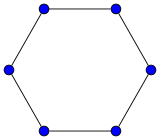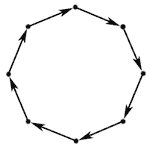# Cycle graph

Cycle graphA cycle graph of length 6
Vertices n
Edges n
Girth n
Automorphisms 2n (Dn)
Chromatic number 3 if n is odd
2 if n is even
Chromatic index 3 if n is odd
2 if n is even
Spectrum {2 cos(2 k π / n); k=1, ... ,n}
Properties 2-regular
Vertex-transitive
Edge-transitive
Unit distance
Hamiltonian
Eulerian
NotationIn graph theory, a cycle graph or circular graph is a graph that consists of a single cycle, or in other words, some number of vertices connected in a closed chain. The cycle graph with n vertices is called Cn. The number of vertices in Cn equals the number of edges, and every vertex has degree 2; that is, every vertex has exactly two edges incident with it.

## Terminology

There are many synonyms for "cycle graph". These include simple cycle graph and cyclic graph, although the latter term is less often used, because it can also refer to graphs which are merely not acyclic. Among graph theorists, cycle, polygon, or n-gon are also often used. A cycle with an even number of vertices is called an even cycle; a cycle with an odd number of vertices is called an odd cycle.

## Properties

A cycle graph is:

• As cycle graphs can be drawn as regular polygons, the symmetries of an n-cycle are the same as those of a regular polygon with n sides, the dihedral group of order 2n. In particular, there exist symmetries taking any vertex to any other vertex, and any edge to any other edge, so the n-cycle is a symmetric graph.

## Directed cycle graphA directed cycle graph is a directed version of a cycle graph, with all the edges being oriented in the same direction.

In a directed graph, a set of edges which contains at least one edge (or arc) from each directed cycle is called a feedback arc set. Similarly, a set of vertices containing at least one vertex from each directed cycle is called a feedback vertex set.

A directed cycle graph has uniform in-degree 1 and uniform out-degree 1.

Directed cycle graphs are Cayley graphs for cyclic groups (see e.g. Trevisan).Wikimedia Commons has media related to Cycle graphs.